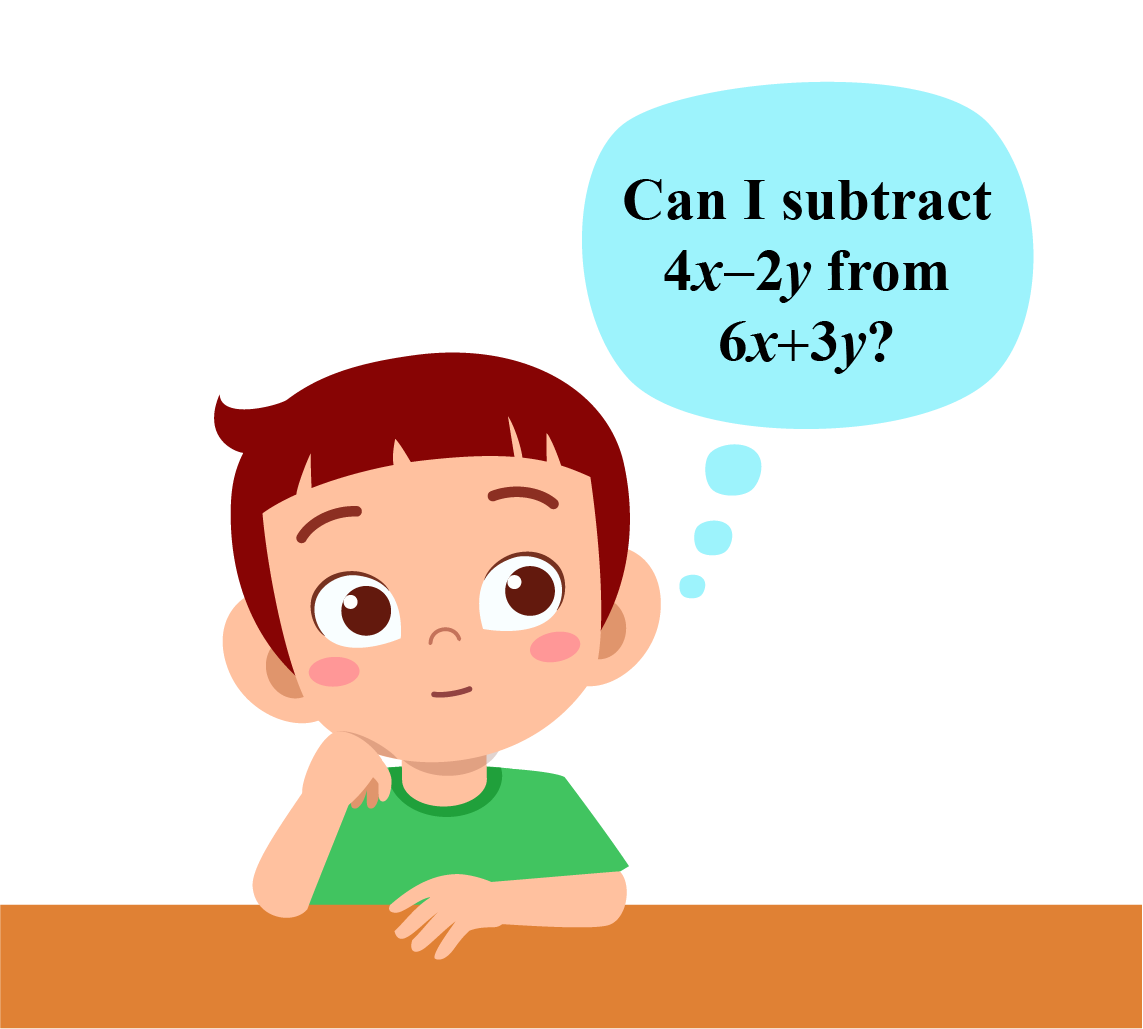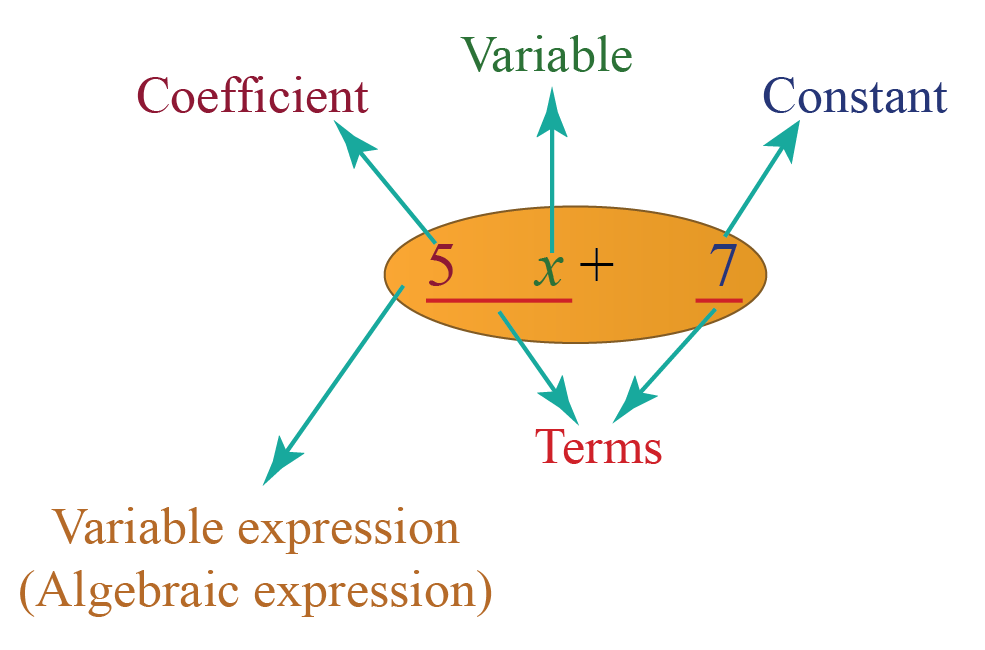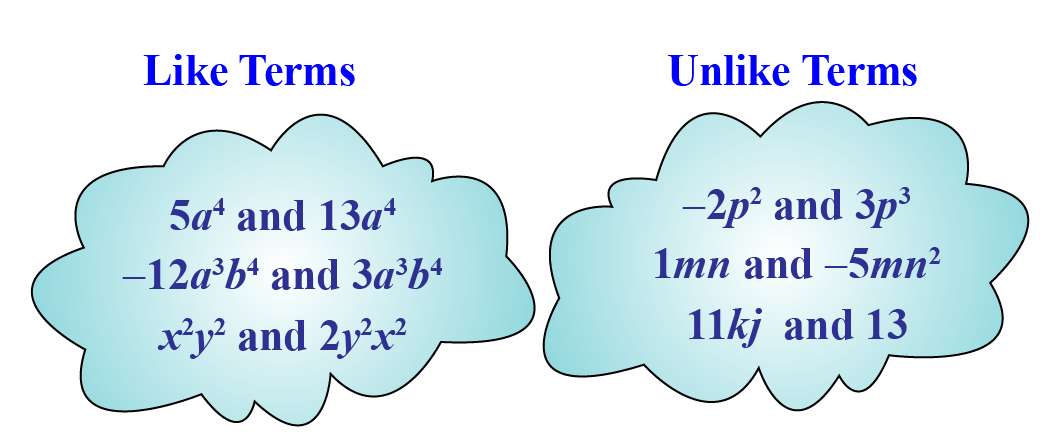# Subtraction of Algebraic Expressions

Subtraction of Algebraic Expressions

Jamie recently learned about algebraic expressions and is now wondering if he can subtract those expressions in the same way he subtracts two whole numbers or fractions.Do you want to help Jamie in subtracting any two or more algebraic expressions?

Let's find out how to subtract algebraic expressions.

In this mini-lesson, we will explore subtraction of algebraic expressions for grade 7 by learning about like and unlike terms, methods to subtract algebraic expressions with the help of interesting simulation, some solved examples and a few interactive questions for you to test your understanding.

## Lesson Plan

 1 How Do You Subtract Algebraic Expressions? 2 Important Notes 3 Solved Examples 4 Challenging Questions 5 Interactive Questions

## What Do You Mean by Like and Unlike Terms?

Before learning about like and unlike terms in an algebraic expression, let us first briefly understand the meaning of algebraic expression.

An algebraic expression (or) a variable expression is a combination of terms by operations such as addition, subtraction, multiplication, division, etc.

An example of an algebraic expression is $$5x+7$$.We can categorize the terms in an algebraic expression into two types - like and unlike terms.

Terms containing same variable raised to the same power are known as like terms.

In like terms, one can only change the numerical coefficient

Examples of like terms are

$$5x$$ and $$13x$$

$$7y^3$$ and $$3y^3$$

$$a^4$$ and $$6a^4$$

in the above examples, only the numerical coefficient differs and we have same variable in each pair of like terms raised to the same power.

Terms that have different variable or same variable raised to different powers are known as unlike terms.

Examples of unlike terms are

$$5x$$ and $$5y$$

$$2m^5$$ and $$8m^3$$

$$56p^3$$ and $$q^3$$

Let us go back to our first example, $$5x+7$$.

This expression has 2 terms, $$5x$$ and $$7$$.

Are these like terms or unlike terms?

$$5x$$ and $$7$$ are unlike terms as they do not have a common variable. First term $$5x$$ has variable $$x$$, while second term $$7$$ does not have any variable. So these are unlike terms.## How Do You Subtract Algebraic Expressions?

Can we subtract $$3$$ apples from $$4$$ bananas?The answer is NO.

We cannot subtract $$3$$ apples from $$4$$ bananas, as they are two different objects.

Similarly, in case of terms in an algebraic expression, we cannot subtract two or more unlike terms.

An important point to remember while subtracting algebraic expressions is that we can only subtract like terms.

There are two methods to do subtraction of algebraic expressions - horizontal method and column method.

### Horizontal Method

Steps to be followed while doing algebraic expression subtraction by horizontal method is written below:

 Step 1- Write all the expressions in a horizontal line by putting them into brackets and put subtraction sign in between. Step 2- Open the brackets. Change the operators whenever there is a negative sign outside the bracket, for example, $$+$$ to $$-$$ and $$-$$ to $$+$$. Step 3- Group all the like terms together from all the expressions and rewrite it in a single expression. Step 4- Add numerical coefficients of all the like terms followed by the common variable. Step 5- Rewrite the simplified expression, and make sure all the terms in the final answer are unlike terms.

### Column Method

Steps to be followed to do subtraction of algebraic expressions by column method is written below:

 Step 1- Write both expressions one below the other. Make sure you have written like terms in one column. \begin{align}2x^2+3x-4y+7 \end{align}            \begin{align}5x+4y-3 \end{align}             If there is a term whose like term is not there in the second expression, for example, $$2x^2$$, then either write $$0$$ below it or leave that column blank. Step 2- Change the operators in the last row (second expression), for example, $$+$$ to $$-$$ and $$-$$ to $$+$$. \begin{align}2x^2+3x-4y+7 \end{align}          \begin{align} \pm 5x\pm 4y\mp 3 \end{align} Step 3- Consider the changed signs and add the numerical coefficient of each column (like terms) and write below it in the same column followed by the common variable. \begin{align}2x^2+3x-4y+7 \end{align} \begin{align} \underline{\ \ \ \ \ \ \ \pm 5x\pm 4y\mp 3} \end{align} \begin{align} 2x^2-2x-8y+10 \end{align} Step 4- Rewrite the final answer. Ignore writing $$0$$ as a term in the final answer, if any.

### Subtraction of Algebraic Expressions Calculator

Try your hands at this simulation of a calculator for subtracting algebraic expressions. Write both the expressions that you want to subtract and click on "Subtract" to get your answer.

More Important Topics
Numbers
Algebra
Geometry
Measurement
Money
Data
Trigonometry
Calculus